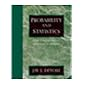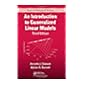Dr. S. R. Lasker Library Online Catalogue

Normal view

# An introduction to generalized linear models / Annette J. Dobson, Adrian G Barnett.

Material type:TextLanguage: English Series: Texts in statistical sciencePublication details: c2008. Edition: 3rd edDescription: 307 p. : ill. ; 24 cmISBN: 9781584889502 (alk. paper); 1584889500 (alk. paper)Subject(s): Linear models (Statistics)DDC classification: 519.5 LOC classification: QA276 | .D589 2008Online resources: Table of contents only | WorldCat Details | E-book Fulltext
Contents:
TOC Model fitting -- Exponential family and generalized linear models -- Estimation -- Inference -- Normal linear models -- Binary variables and logistic regression -- Nominal and ordinal logistic regression -- Poisson regression and log-linear models -- Survival analysis -- Clustered and longitudinal data -- Bayesian analysis -- Markov chain Monte Carlo methods -- Example Bayesian analyses.
Summary: Summary: Offers a cohesive framework for statistical modeling. Emphasizing numerical and graphical methods, this work enables readers to understand the unifying structure that underpins GLMs. It discusses common concepts and principles of advanced GLMs, including nominal and ordinal regression, survival analysis, and longitudinal analysis.
Tags from this library: No tags from this library for this title.
Star ratingsAverage rating: 0.0 (0 votes)
Holdings
Item type Current library Collection Call number Copy number Status Date due Barcode Item holds Course reservesE-Book
E-book
Non-fiction 519.5 DOI 2008 (Browse shelf(Opens below)) Not For LoanText
Reserve Section
Non-fiction 519.5 DOI 2008 (Browse shelf(Opens below)) C-1 Not For Loan 25696Text
Reserve Section
Non-fiction 519.5 DOI 2008 (Browse shelf(Opens below)) C-2 Checked out Not For Loan 19/01/2023 25698Text
Circulation Section
Non-fiction 519.5 DOI 2008 (Browse shelf(Opens below)) C-3 Available 26760Text
Circulation Section
Non-fiction 519.5 DOI 2008 (Browse shelf(Opens below)) C-4 Available 26761
Total holds: 0
##### Browsing EWU Library shelves, Shelving location: Circulation Section Close shelf browser (Hides shelf browser)519.5 DEP 2004 Probability and statistics for engineering and the sciences / 519.5 DEP 2004 Probability and statistics for engineering and the sciences / 519.5 DOI 2008 An introduction to generalized linear models / 519.5 DOI 2008 An introduction to generalized linear models / 519.5 FRS 1991 Statistics : 519.5 HOI 2005 Introduction to mathematical statistics / 519.5 HOI 2005 Introduction to mathematical statistics /

Includes bibliographical references (p. -301) and index.

TOC Model fitting --
Exponential family and generalized linear models --
Estimation --
Inference --
Normal linear models --
Binary variables and logistic regression --
Nominal and ordinal logistic regression --
Poisson regression and log-linear models --
Survival analysis --
Clustered and longitudinal data --
Bayesian analysis --
Markov chain Monte Carlo methods --
Example Bayesian analyses.

Summary:
Offers a cohesive framework for statistical modeling. Emphasizing numerical and graphical methods, this work enables readers to understand the unifying structure that underpins GLMs. It discusses common concepts and principles of advanced GLMs, including nominal and ordinal regression, survival analysis, and longitudinal analysis.

AS

Tahur Ahmed

There are no comments on this title.